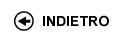Politecnico di Torino
03KXTLZ, 03KXTJM, 03KXTLI, 03KXTLM, 03KXTLP, 03KXTLS, 03KXTLX, 03KXTMA, 03KXTMB, 03KXTMC, 03KXTMH, 03KXTMK, 03KXTMO, 03KXTMQ, 03KXTNX, 03KXTOD, 03KXTPC, 03KXTPI, 03KXTPL
Linear algebra and geometry
Corso di Laurea in Ingegneria Aerospaziale - Torino
Corso di Laurea in Ingegneria Meccanica (Mechanical Engineering) - Torino
Corso di Laurea in Ingegneria Dell'Autoveicolo (Automotive Engineering) - Torino
Espandi...
 Docente Qualifica Settore Lez Es Lab Tut Anni incarico Carlini EnricoO2 MAT/03 60 30 10 0 6 Vaccarino FrancescoA2 MAT/03 60 30 10 0 3
 SSD CFU Attivita' formative Ambiti disciplinari MAT/03MAT/08 73 A - Di baseA - Di base Matematica, informatica e statisticaMatematica, informatica e statistica
Esclusioni:
01RKC
 Presentazione The course has two main goals. The first one is to introduce the main topics of linear algebra and geometry, training the student to follow logical deductive arguments and to use the proper formal language. The second goal is to give to the students the main concepts of some basic numerical methods of linear algebra and of MATLAB programming language, which is by now widely used in engineering. The student will learn to tackle and solve some linear algebra problems, that generally occur as intermediate steps in the solution of more complex mathematical models for engineering problems and that cannot be solved by analytical means. Risultati di apprendimento attesi The student will be able to follow proofs, to build examples and counterexamples and to use the basic properties of analytic geometry and of vector spaces. The student will be able to work with matrices and to solve systems of linear equations. Moreover, given a mathematical problem of linear algebra (e.g. solution of a linear system or computation of eigenvalues of a matrix), the student will be able to identify and to apply (using MATLAB) the most efficient numerical method among the presented ones, which gives an approximation of the solution with the best possible accuracy and with the lowest computational cost. Prerequisiti / Conoscenze pregresse A working knowledge of the mathematical tools presented in the first semester. In particular, a basic knowledge of real and complex numbers, solving equations and inequalities of degree one or two, differential and integral calculus as given in Mathematical Analysis 1, as well as the main syntactic constructs used in computer programming, taught in the course of Computer Sciences. Programma • Vector in 2-space and in 3-space and their operations. Dot product, cross product and box product. Lines and planes in 3-space. Orthogonal projections. • Matrices and their operations. Strongly reduced matrices. Matrix form of linear systems of equations and their solutions with geometrical applications. Matrix equations and inverse of a matrix. Determinants. • Vector spaces: definition, examples and applications. Sub-vector spaces and main operations with them. • Linear combination and linearly dependent vectors. How to extract linearly independent vectors from a set. Bases of a vectors space. Dimension of a vector space. Dimension of finitely generated subspace. • Space of polynomials. Grassmann's relation. • Linear maps. Image of a linear map. Injective and surjective linear maps. Isomorphisms. • Matrix of a linear map. Endomorphism and square matrices. • Eigenvalues and eigenvectors. Eigenspaces of matrix endomorphisms. Characteristic polynomial of an endomorphism. Diagonalization of and endomorphism. • Orthonormal bases, orthonormal matrices. Gram-Schmidt's algorithm. Diagonalization of real symmetric matrices using orthogonal matrices. • Metric problems: distance between two points, two lines, and a point and a line. • Quadratic geometry: conic curves, and spheres. Non-degenerate quadrics in canonical form. Recognising a quadric surface. • General concepts on numerical problems and algorithms. Machine numbers, rounding error. • Approximation of functions and numerical data. Polynomial interpolation: Lagrange and Newton representations. Choice of interpolation points and convergence properties. Piecewise polynomial interpolation: splines. Overdeterminated and underdeterminated linear systems. • Numerical solution of linear systems. Matrix norms and condition number of a linear system. Substitution technique of triangular linear systems. Gauss elimination method. Partial pivoting. PA=LU factorization and its applications. Choleski factorization and its applications. QR factorization. Least squares method. • Numerical computation of eigenvalues. Power method and inverse power method. QR method. Singular value decomposition and its applications. Organizzazione dell'insegnamento Exercises will cover the topics of the lectures. Some will be carried out by the teacher at the blackboard, others will actively involve the students. Moreover, computer room activities are also scheduled, during which the numerical algorithms presented in the lectures will be implemented by using the MATLAB software. The application of these algorithms will allow to study in a deeper way the theoretical aspects and the properties of the presented numerical methods, and to perform a critical analysis of the numerical results. Testi richiesti o raccomandati: letture, dispense, altro materiale didattico L. Gatto, Lezioni di Algebra lineare e Geometria, Clut 2013. S.Greco, P. Valabrega, Lezioni di Geometria, Vol. 1 Algebra lineare, Vol. 2 Geometria Analitica, Ed. Levrotto e Bella, Torino 2009. E.Carlini, 50quiz di Geometria, Celid 2011. G. Casnati, M.L. Spreafico, Allenamenti di Geometria, Ed. Esculapio, Bologna 2013. J. Cordovez, Chissŕ chi lo sa?, Clut 2013. G. Monegato, Metodi e algoritmi per il Calcolo Numerico, CLUT 2008. L. Scuderi, Laboratorio di Calcolo Numerico. Esercizi di Calcolo Numerico risolti con Matlab, CLUT 2005. Further course material as lectures on-line, lecture notes, proposed and solved exercises will be given through the ‘portale della didattica’ website. Criteri, regole e procedure per l'esame The exam will cover theoretical and applied aspects. There will be a written part and a LAIB part. During the exam it is strictly forbidden to use any kind of communication devices. More details will be given during the lectures and on the web page of the course. Orario delle lezioni Statistiche superamento esami Programma definitivo per l'A.A.2016/17